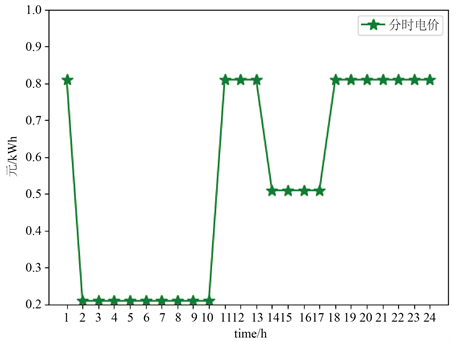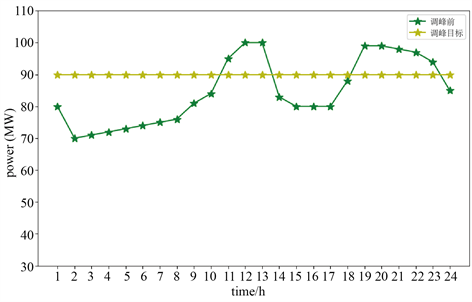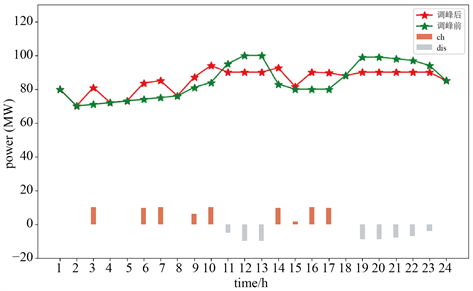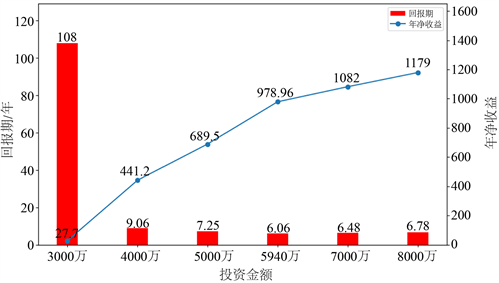﻿ 获取多场景收益的电网侧储能容量优化配置

# 获取多场景收益的电网侧储能容量优化配置Optimal Allocation of Grid-Side Energy Storage Capacity to Obtain Multi-Scenario Benefits

Abstract: Due to its rapid adjustment and flexibility, energy storage systems will soon become an important part of the power system. Although the cost has been reduced, the single application scenario of the energy storage system is still difficult to make profits effectively or recover the cost in the short term. Therefore, the optimal allocation of energy storage capacity has gradually attracted the attention of the industry. In view of the current grid energy storage system, application scenario is relatively single, we propose a grid side energy storage capacity allocation method that takes into account the superlinear benefits of peak regulation auxiliary services combined with TOU (Time of Use), to consider energy storage building investment and operational cost of peak shaving, peak valley arbitrage profits, the delay of benefit maximization as the objective function, such as network equipment upgrades the energy storage capacity of the optimizing configuration model is constructed. Finally, based on the grid data of Xinjiang region, the optimal energy storage rated power and capacity configuration results are obtained through Python-Gurobi simulation calculation, and the economic indicators are analyzed to verify the effectiveness and feasibility of the method, which provides advice for the investment of grid side energy storage projects.

1. 引言

2. 结合分时电价的调峰辅助服务收益架构

2.1. 考虑延缓变压器升级的调峰服务模型

${y}_{t}={R}_{t}^{dis}\cdot {\varpi }_{ht}-\left({C}_{t}^{peak}-{R}_{t}^{dis}\right)\cdot 2\cdot {\varpi }_{ht},0\le {R}_{t}^{dis}\le {C}_{t}^{peak}$ (1)

${y}_{t}={C}_{t}^{peak}\cdot {\varpi }_{ht},{C}_{t}^{peak}\le {R}_{t}^{dis}\le {R}_{\mathrm{max}}$ (2)

${K}_{1}=\underset{t=1}{\overset{T}{\sum }}{y}_{t}$ (3)

${K}_{2}=r\cdot C\cdot \eta \cdot {R}^{rate}$ (4)

${K}_{2}$ 为储能系统延缓设备投资效益，元；r为输配电设备的固定折旧率；C为输配电设备的单位容量造价，元/MW； $\eta$ 为考虑并网设备与储能设备充放电损耗的储能效率； ${R}^{rate}$ 储能设备的最大功率，MW。

2.2. 峰谷套利模型Figure 1. Xinjiang Region TOU electricity price schematic diagram

${K}_{1}=\underset{t\in T}{\sum }{\varpi }_{t}\cdot \left({R}_{t}^{dis}-{R}_{t}^{ch}\right)\cdot \Delta t$ (5)

3. 储能全寿命周期成本模型

3.1. 储能建造投资成本

BESS的初始投资建造成本通常包括换流器建造成本和电池容量成本。初始投资建造成本指储能工程投建初期一次性投入的固定资金。全寿命周期内BESS的投资成本如式(6)所示。

${C}_{inv}={R}^{rate}\cdot {\varpi }_{{R}_{inv}}+E\cdot {\varpi }_{{E}_{inv}}$ (6)

$AF=\frac{r{\left(1+r\right)}^{L}}{{\left(1+r\right)}^{L}-1}$ (7)

AF为年金因子，无量纲；r为折现率，默认为8%；L为使用年限，年。

3.2. 运行维护成本

1) 可变运行费用

${\varpi }_{b}=\frac{{\varpi }_{cell}\cdot {10}^{6}}{2{N}_{cycle}\cdot \left(SO{C}_{\mathrm{max}}-SO{C}_{\mathrm{min}}\right)}$ (8)

${C}_{de}\left({R}_{t}^{dis},{R}_{t}^{ch}\right)={\varpi }_{b}\cdot \underset{t=1}{\overset{T}{\sum }}\left({R}_{t}^{dis}+{R}_{t}^{ch}\right)\cdot \Delta t$ (9)

${C}_{de}\left({R}_{t}^{dis},{R}_{t}^{ch}\right)$ 为电池容量退化成本，元，T为24。

${C}_{om}={C}_{fix}+{C}_{var}={R}^{rate}\cdot {\varpi }_{{R}_{om}}^{F}+365\ast {C}_{de}\left({R}_{t}^{dis},{R}_{t}^{ch}\right)$ (10)

${C}_{om}$ 为储能设备的运行维护成本，元； ${\varpi }_{{R}_{om}}^{F}$ 为单位电池储能每天固定功率运行维护成本，元/(MW·年)； ${R}^{rate}$ 为节点i上电池储能功率，MW。

4. 获取多场景收益的储能容量配置模型

4.1. 目标函数

$\mathrm{max}f=\mathrm{max}\left(\left({K}_{1}+{K}_{3}\right)\cdot 365+AF\cdot {K}_{2}-AF\cdot {C}_{inv}-{C}_{om}\right)$ (11)

4.2. 约束条件

${e}_{t}={e}_{t-1}+\Delta t\cdot {R}_{t}^{ch}\cdot {\eta }_{ch}-\Delta t\cdot {R}_{t}^{dis}/{\eta }_{dis}$ (12)

SOC上下限约束：

$SO{C}^{\mathrm{min}}\cdot E\le {e}_{t}\le SO{C}^{\mathrm{max}}\cdot E$ (13)

${e}_{0}={e}_{T}$ (14)

$0\le {R}_{t}^{ch}\le {x}_{1,t}\cdot {R}^{rate}$ (15)

$0\le {R}_{t}^{dis}\le {x}_{2,t}\cdot {R}^{rate}$ (16)

${x}_{1,t}+{x}_{2,t}\le 1$ (17)

${R}^{\mathrm{min}}\le {R}^{rate}\le {R}^{\mathrm{max}}$ (18)

${E}^{\mathrm{min}}\le E\le {E}^{\mathrm{max}}$ (19)

${R}_{i}^{\mathrm{max}}$${R}_{i}^{\mathrm{min}}$ 为待安装储能的功率上下限； ${E}^{\mathrm{max}}$${E}^{\mathrm{min}}$ 分别为待安装储能的容量上下限。

${R}^{rate}\cdot {\varpi }_{{R}_{inv}}+E\cdot {\varpi }_{{E}_{inv}}\le \gamma$ (20)

${\varpi }_{{R}_{inv}}$ 为单位电池储能功率建设成本，元/MW； ${R}^{rate}$ 为待建的电池储能最大功率，MW； ${\varpi }_{{E}_{inv}}$ 为电池储能的单位容量价格，元/MWh；E为待建的电池储能装置容量，MWh

4.3. 模型线性化

${R}_{t}^{dis}={k}_{1,t}\cdot 0+{k}_{2,t}\cdot {C}_{t}^{peak}+{k}_{3,t}\cdot {R}_{\mathrm{max}}$ (21)

${y}_{t}={k}_{1,t}\cdot \left(-2{C}_{t}^{peak}\cdot {\varpi }_{ht}\right)+{k}_{2,t}\cdot {C}_{t}^{peak}\cdot {\varpi }_{ht}+{k}_{3,t}\cdot {C}_{t}^{peak}\cdot {\varpi }_{ht}$ (22)

${k}_{1,t}\le {z}_{1,t},{k}_{2,t}\le {z}_{1,t}+{z}_{2,t},{k}_{3,t}\le {z}_{2,t}$ (23)

${k}_{1,t}+{k}_{2,t}+{k}_{3,t}=1$ (24)

${z}_{1,t}+{z}_{2,t}=1$ (25)

${R}_{1,t}={x}_{1,t}\cdot {R}^{rate}$ (26)

${R}_{1,t}\le {x}_{1,t}\cdot {R}_{\mathrm{max}}$ (27)

${R}_{1,t}\le {R}^{rate}$ (28)

${R}_{1,t}\ge {R}^{rate}-{R}_{\mathrm{max}}\cdot \left(1-{x}_{1,t}\right)$ (29)

${R}_{2,t}={x}_{2,t}\cdot {R}^{rate}$ (30)

${R}_{2,t}\le {x}_{2,t}\cdot {R}_{\mathrm{max}}$ (31)

${R}_{2,t}\le {R}^{rate}$ (32)

${R}_{2,t}\ge {R}^{rate}-{R}_{\mathrm{max}}\cdot \left(1-{x}_{2,t}\right)$ (33)

5. 算例分析

5.1. 数据说明

5.2. 算例结果分析

1) 储能优化配置结果Figure 2. Schematic diagram of peak regulation before and target of peak regulationTable 1. Revenue parameters related to peak regulationTable 2. Optimal configuration results of energy storage

2) 优化模型的有效性分析(从充放电行为、削峰填谷效果进行分析)Figure 3. Comparison diagram before and after peak regulation and energy storage charging and discharging behavior

3) 初始投资总额限制对于收益以及初始回报期的影响Table 3. Influence of investment amount on energy storage allocationFigure 4. The influence of investment amount change on annual net income and return period

4) 储能成本变化对储能投资商的影响Table 4. The impact of energy storage investment cost reduction on energy storage project investment

6. 结论与展望

1) 本文分析了新疆地区的调峰辅助服务以及该地区的分时电价分布特点，提出考虑分时电价政策的储能调峰辅助服务超线性收益理论，该理论对于指导储能投资商投资、管理项目有一定的指导作用。

2) 基于第一点提出的考虑分时电价政策的储能多场景收益理论，搭建了对应的模型，通过gurobi + python进行仿真计算，证明了所提模型的合理性。与此同时，算例分析了投资金额限制以及未来阶段储能电池价格大幅度下降对该多场景收益模型的影响，算例说明，一方面投资金额在某一数值时，项目能在最短时间内回收成本，另一方面，未来储能价格下降会导致回收周期缩短，甚至改变储能盈利的重点。

NOTES

*通讯作者。

 王敏. 我国可再生能源发展面临挑战[N]. 社会科学报, 2019-11-14(002).

 赖昌伟, 黎静华, 陈博, 黄玉金, 韦善阳. 光伏发电出力预测技术研究综述[J]. 电工技术学报, 2019, 34(6): 1201-1217.

 鲁宗相, 李海波, 乔颖. 高比例可再生能源并网的电力系统灵活性评价与平衡机理[J]. 中国电机工程学报, 2017, 37(1): 9-20.

 葛晓琳, 金言, 夏澍, 符杨. 面向调峰调频需求的风水火电协调优化调度[J]. 电网技术, 2019, 43(11): 3917-3926.

 刘冰, 张静, 李岱昕, 等. 储能在发电侧调频调峰服务中的应用现状和前景分析[J]. 储能科学与技术, 2016, 5(6): 909-914.

 Zakeri, B. and Syri, S. (2015) Electrical Energy Storage Systems: A Comparative Life Cycle Cost Analysis. Renewable & Sustainable Energy Reviews, 42, 569-596.
https://doi.org/10.1016/j.rser.2014.10.011

 王良缘, 江岳文, 王杰. 考虑参与多市场交易的电网侧储能优化配置[J]. 电网与清洁能源, 2020, 36(11): 30-38.

 马美婷, 袁铁江, 陈广宇, 蔡高雷, 彭生江, 张增强. 储能参与风电辅助服务综合经济效益分析[J]. 电网技术, 2016, 40(11): 3362-3367.

 梁亮, 李建林, 惠东. 大型风电场用储能装置容量的优化配置[J]. 高电压技术, 2011, 37(4): 930-936.

 刘皓明, 陆丹, 杨波, 姚艳, 叶季蕾, 薛金花. 可平抑高渗透分布式光伏发电功率波动的储能电站调度策略[J]. 高电压技术, 2015, 41(10): 3213-3223.

 南国良, 张露江, 郭志敏, 何洋, 刘萌, 秦嘉翼, 姜欣. 电网侧储能参与调峰辅助服务市场的交易模式设计[J]. 电气工程学报, 2020, 15(3): 88-96.

 路小俊, 伊建伟, 李炎. 基于多目标网格自适应搜索算法的储能系统参与AGC优化控制策略[J]. 电网技术, 2019, 43(6): 2116-2124.

 Zhang, Y., Xu, Y., Yang, H., et al. (2019) Optimal Whole-Life-Cycle Planning of Battery Energy Storage for Multi-Functional Services in Power Systems. IEEE Transactions on Sustainable Energy, 11, 2077-2086.

 黎静华, 汪赛. 兼顾技术性和经济性的储能辅助调峰组合方案优化[J]. 电力系统自动化, 2017, 41(9): 44-50+150.

 Oudalov, A., Chartouni, D., Ohler, C., et al. (2007) Value Analysis of Battery Energy Storage Applications in Power Systems. IEEE Power Systems Conference & Exposition, Atlanta, 29 October-1 November 2006, 2206-2211.
https://doi.org/10.1109/PSCE.2006.296284

 黄碧斌, 胡静, 蒋莉萍, 李琼慧, 冯凯辉, 元博. 中国电网侧储能在典型场景下的应用价值评估[J/OL]. 中国电力: 1-8.
https://kns-cnki-net.webvpn2.ncepu.edu.cn/kcms/detail/11.3265.tm.20200806.1807.008.html, 2021-03-11.

 Ortega-Vazquez, M.A. (2014) Optimal Scheduling of Electric Vehicle Charging and Vehicle-to-Grid Services at Household Level Including Battery Degradation and Price Uncertainty. IET Generation Transmission & Distribution, 8, 1007-1016.
https://doi.org/10.1049/iet-gtd.2013.0624

 Xu, B.L., et al. (2016) Modeling of Lithium-Ion Battery Degradation for Cell Life Assessment. IEEE Transactions on Smart Grid, 9, 1131-1140.

Top